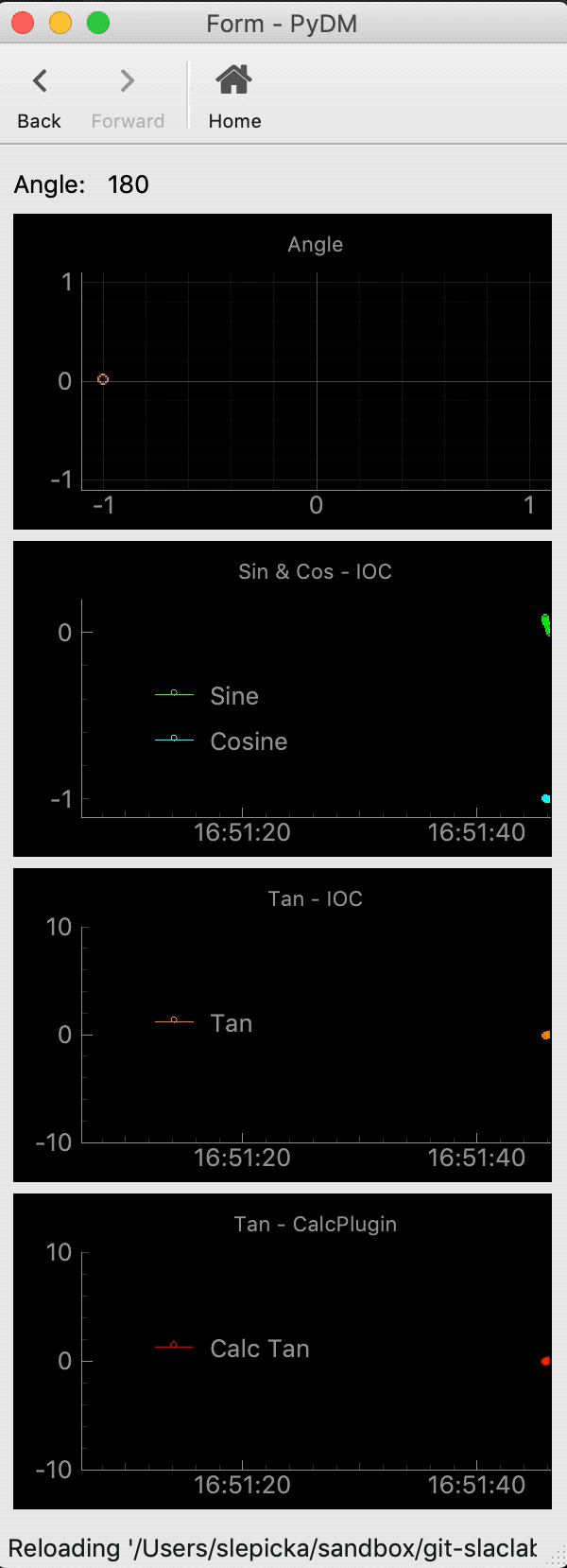# Calc Plugin

PyDM uses Data Plugins as sources of information to be displayed at the widgets. Calc Plugin allows users to create and use mathematical expressions.

The Calc Plugin takes in data from given channels and then applies a mathematical expression, the result is broadcast to all the listeners connected to this particular calc channel.

By default, as soon as any widgets gets connected to the same channel variable, they will get the results from the mathematical expression defined in the channel’s address. These widgets can receive any updates when the calc channel’s output changes. For example, if the user has added a calc channel to a PyDMLabel, then the PyDMLabel will update whenever a new value from one of the channels listed by the calc plugin is pushed to the calc plugin.

General Calc Plugin channel syntax:

```calc://my_variable_name?expr_var_name=channel://address&expr_var_name_two=channel://address&expr=math expression
```

Note

Once a calc channel is created, multiple widgets can be connected to the same channel by providing the name of the variable, like so:

```calc://my_variable_name
```

Note

The calc functions uses url formatting. Where the name attribute is separated by the ? symbol and all other attributes are separated by the & symbol.

## Required Attributes

In order to be able to properly create a calc channel, all the required attributes must be provided in the channel’s address.

The table below explains the attributes that are required to create a local plugin channel:

Attributes

Description

Format Example

calc

protocol name for Calc Plugin

calc://

name

the identifier for the mathematical expression
user’s choice

my_expr_name

var

variable mapped to an address for the expr
attribute.

expr

mathematical expression

expr=math expression

Here is a simple example of a channel address format with the required attributes:

```calc://circ?var=ca://DEMO:ANGLE&expr=math.sin(math.radians(var))
```

### Extra Attributes

Along with the required attributes, the Local Data Plugin can also accept some optional attributes to configure the Local Variables with. These attributes should be provided in the “extras” dictionary. The optional attributes are described in the extra attributes table below:

The table below explains the optional attributes that can go in the extras:

Attributes

Description Type

Format Example

update

The calc function will update when one of the
variables in the update list receives a new
value optional. If nothing is given, the calc
function will run anytime one of the variables

update=var, var_two

Note

The “extras” Attributes are all optional, any number of desired attributes can be specified, or none.

Here is a simple example of a channel address format with some optional attributes:

```calc://circ?var=ca://DEMO:ANGLE&var_two=loc://int_var&expr=var_two*var&update=var_two
```

### Built-in Calc Helpers

Certain helper functions are built in to PyDM because they get semi-frequent use in the context of EPICS values.

Helpers

Description

Usage Example

epics_string

Convert a char waveform to a string.

calc://my_string?var=ca://WAVEFORM:PV&expr=epics_string(var)

epics_unsigned

Force a signed integer to be unsigned.

calc://my_int?var=ca://SOME:16BIT:INT&expr=epics_unsigned(var, 16)

You should use epics_string when you have a string PV that is expressed as a char waveform, but you need to use the corresponding string value internally.

You should use epics_unsigned when you are dealing with a PV that is supposed to be interpreted as a positive integer but is instead a negative integer because channel access does not support any unsigned types and we have overflowed to negative values.

### Simple Calc Plugin Example

The picture below represents an example of using the Calc Plugin. Calc addresses given in the channels of the Wavefrom Curve Editor of a PYDMWavefromPlot:

```calc://circleX?angle=ca://DEMO:ANGLE&expr=-1*math.cos(math.radians(180-angle))
```

Calc address given in the channels of the Wavefrom Curve Editor of a PYDMWavefromPlot to get the Tangent:

```calc://tanval?angle=ca://DEMO:ANGLE&expr=tan(radians(angle)) if angle not in [90, 270] else None
```

Values for TAN### Miscellaneous

• See https://docs.python.org/3/library/math.html for mathematical operations which can be used in the given expression.

• NumPy is a valid library for the mathematical expression and can be accessed via ‘numpy.xyz’ or ‘np.xyz’.

• Already established local variables can be used in a calc variable attribute, but it is not possible to create a local plugin variable inside a calc variable attribute.

• The calc plugin is intended to be only one level deep and will break if a calc channel is set as a variable of another calc channel.